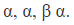×
Get Full Access to Introductory Chemistry - 5 Edition - Chapter 17 - Problem 68p
Get Full Access to Introductory Chemistry - 5 Edition - Chapter 17 - Problem 68p

×

# Write a partial decay series for Rn-220 undergoing these sequential decays.?, ?, ? ?ISBN: 9780321910295 34

## Solution for problem 68P Chapter 17

Introductory Chemistry | 5th Edition

• Textbook Solutions
• 2901 Step-by-step solutions solved by professors and subject experts
• Get 24/7 help from StudySoup virtual teaching assistantsIntroductory Chemistry | 5th Edition

4 5 1 364 Reviews
17
0
Problem 68P

Problem 68P

Write a partial decay series for Rn-220 undergoing these sequential decays.

α, α, β α.

Step-by-Step Solution:

Problem 68P

Write a partial decay series for Rn-220 undergoing these sequential decays.Step by Step Solution

Step 1 of 2

A nuclear equation represents changes that occur during nuclear processes such as radioactivity.

To balance the equation, the sum of the atomic number on both sides of the equation must be equal and the sum of the mass mass number on both sides of the equation must also be equal.

Step 2 of 2

## Discover and learn what students are asking

Statistics: Informed Decisions Using Data : Introduction to the Practice of Statistics
?Explain the difference between a population and a sample.

Chemistry: The Central Science : Periodic Properties of the Elements
?Give three examples of ions that have an electron configuration of $$n d^{6}(n=3,4,5, \ldots)$$.

Unlock Textbook Solution